International
Tables for
Crystallography
Volume A
Space-group symmetry
Edited by M. I. Aroyo

International Tables for Crystallography (2015). Vol. A, ch. 1.4, p. 48

## Section 1.4.1.4.8. Cubic space groups

H. Wondratscheke

#### 1.4.1.4.8. Cubic space groups

| top | pdf |

There are five cubic crystal classes combined with the three types of lattices P, F and I in which the cubic space groups are classified. The two symmetry directions  and  are the representative directions in the space groups of the crystal classes 23 and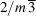. A third representative symmetry direction,, is added for space groups of the crystal classes 432,and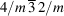.6

In the full HM symbol the symmetry is described as usual. Examples are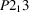,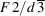,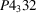,,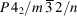and finally No. 230,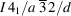. The short HM symbols of the noncentrosymmetric space groups (those of crystal classes 23, 432 and) are the same as the full HM symbols. In the short HM symbols of centrosymmetric space groups of the crystal classesandthe rotations or screw rotations are omitted with the exception of the rotations 3 and rotoinversionswhich represent the symmetry in direction . Thus, in the examples listed above,,andare the short HM symbols differing from the full HM symbols.

As in the orthorhombic space groups I222 and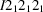, there is the pair I23 and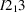in which the `simplest symmetry operation' rule is violated. In both space groups twofold rotations and screw rotations around a, b and c occur simultaneously. In I23 the rotation axes intersect, inthey do not. The first space group can be generated by adding the I-centring to the space group P23, the second is obtained by adding the I-centring to the space group.